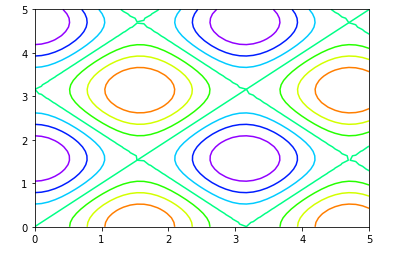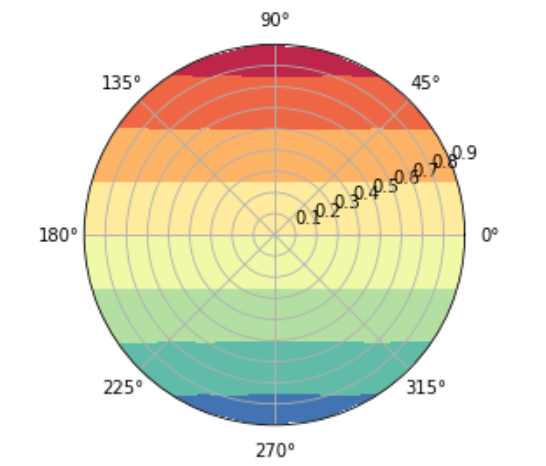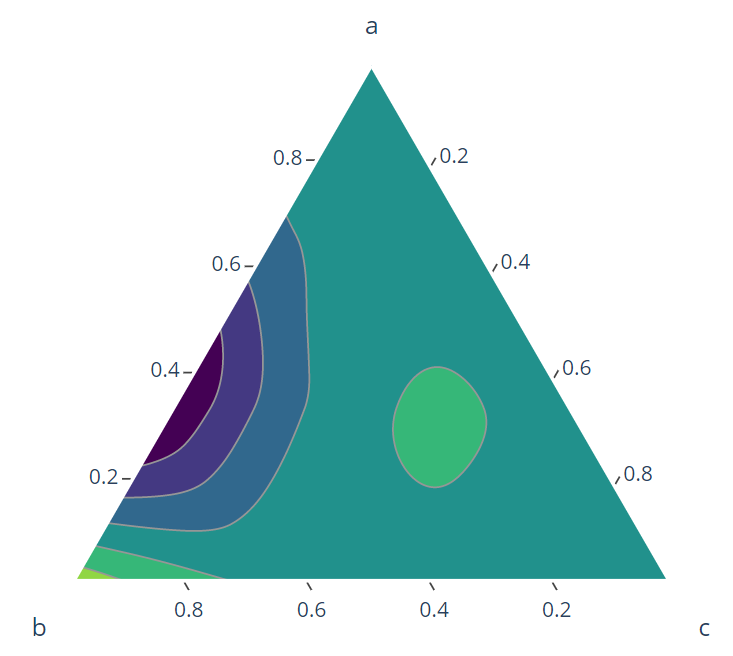# Contour plots

• Last Updated : 22 Jan, 2021

A contour plot is a graphical method to visualize the 3-D surface by plotting constant Z slices called contours in a 2-D format. The contour plot is an alternative to a 3-D surface plot

The contour plot is formed by:

• Vertical axis: Independent variable 2
• Horizontal axis: Independent variable 1
• Lines: iso-response values, can be calculated with the help (x,y).

The independent variable usually restricted to a regular grid. The actual techniques for determining the correct iso-response values are rather complex and almost always computer-generated.

The contour plot is used to depict the change in Z values as compared to X and Y values. If the data (or function) do not form a regular grid, you typically need to perform a 2-D interpolation to form a regular grid.

For one variable data, a run sequence/ histogram is considered necessary. For two-variable data, a scatter plot is considered necessary. The contour plots can also polar co-ordinates (r,theta) instead of traditional rectangular (x, y, z) coordinates.

#### Types of Contour Plot:

• Rectangular Contour plot: A projection of 2D-plot in 2D-rectangular canvas. It is the most common form of the contour plot.
• Polar contour plot: Polar contour plot is plotted by using the polar coordinates r and theta. The response variable here is the collection of values generated while passing r and theta into the given function, where r is the distance from origin and theta is the angle from the positive x axis.
• Ternary contour plot: Ternary contour plot is used to represent the relationship between 3 explanatory variables and the response variable in the form of a filled triangle.

Contour plot can be plotted in different programming languages:

• Python/ Matplotlib: Contour plot can be plotted using plt.contour or plt.contourf functions, where plt is matplotlib.pyplot. The difference between these two that plot.contour generates hollow contour plot, the plt.contourf generated filled.
• Matlab: functions such as contourf (2d-plot) and contour3 (3D-contour) can be used for contour plotting
• R: can create a contour plot with filled.contour functions in R.

#### Implementations:

• Rectangular Contour Plot: Below is the sample code for plotting rectangular contour plots in Python and matplotlib.

## Python3

 `#  imports ` `import` `numpy as np ` `import` `matplotlib.pyplot as plt ` `# ` `# define a function ` `def` `func(x, y): ` `    ``return` `np.sin(x) ``*``*` `2` `+`  `np.cos(y) ``*``*``2` `# generate 50 values b/w 0 a5 ` `x ``=` `np.linspace(``0``, ``5``, ``50``) ` `y ``=` `np.linspace(``0``, ``5``, ``50``) ` ` `  `# Generate combination of grids ` `X, Y ``=` `np.meshgrid(x, y) ` `Z ``=` `func(X, Y) ` ` `  `# Draw rectangular contour plot ` `plt.contour(X, Y, Z, cmap``=``'gist_rainbow_r'``); `• Polar Contour plot: For plotting polar contour plot we need to define first r and theta. Below is the sample code for plotting polar contour plots using matplotlib subplots.

## Python3

 `# generate r and theta arrays ` `rad_arr ``=` `np.radians(np.linspace(``0``, ``360``, ``20``)) ` `r_arr ``=` `np.arange(``0``, ``1``, .``1``) ` `# define function ` `def` `func(r, theta): ` `  ``return` `r ``*` `np.sin(theta)  ` ` `  `r, theta ``=` `np.meshgrid(r_arr, rad_arr) ` `# get the values of response variables ` `values ``=` `func(r,theta) ` ` `  `# plot the polar coordinates ` `fig, ax ``=` `plt.subplots(subplot_kw``=``dict``(projection``=``'polar'``)) ` `ax.contourf(theta, r, values, cmap``=``'Spectral_r'``) ` ` `  `plt.show()`Polar contour plot

• Ternary Contour Plot: Matplotlib does not provide a definitive API for plotting Ternary Contour plot, however, there are many other package which does that. IN this example, we will be using Plotly library.

## Python3

 `# install & import plotly ` `! pip install plotly ` `import` `plotly.figure_factory as ff ` ` `  `# Define variables ` `a ``=` `np.array([``0.` `, ``0.` `, ``0.``, ``0.``, ``1.``/``3``, ``1.``/``3``, ``1.``/``3``, ``2.``/``3``, ``2.``/``3``, ``1.``]) ` `b ``=` `np.array([``0.``, ``1.``/``3``, ``2.``/``3``, ``1.``, ``0.``, ``1.``/``3``, ``2.``/``3``, ``0.``, ``1.``/``3``, ``0.``]) ` `c ``=` `1` `-` `a ``-` `b ` `# Define function that generates response variable ` `func ``=` `(a ``-` `0.02``) ``*` `b ``*` `(a ``-` `0.5``) ``*` `(b ``-` `0.4``) ``*` `(c ``-` `1``)``*``*``2` ` `  `# plot ternary contour ` `fig ``=` `ff.create_ternary_contour(np.array([a, b, c]), func, ` `                                ``pole_labels``=``[``'a'``, ``'b'``, ``'c'``], ` `                                ``interp_mode``=``'cartesian'``, ` `                                ``colorscale``=``'Viridis'``,) ` `fig.show() `Ternary Contour plot

#### References:

My Personal Notes arrow_drop_up
Recommended Articles
Page :